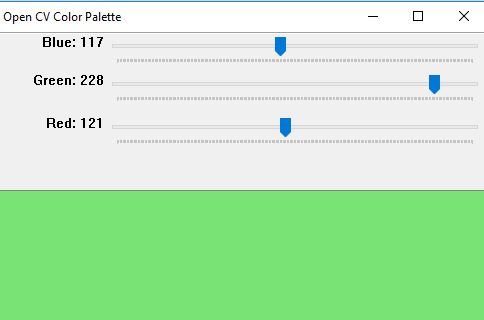# Python | OpenCV BGR color palette with trackbars

• Last Updated : 13 Nov, 2018

OpenCV is a library of programming functions mainly aimed at real-time computer vision.
In this article, Let’s create a window which will contain RGB color palette with track bars. By moving the trackbars the value of RGB Colors will change b/w 0 to 255. So using the same, we can find the color with its RGB values.

```Libraries needed:
OpenCV
Numpy```

Approach:
Create a black window with three color channels with resolution 512 x 512. Then create three ‘B’ ‘G’ ‘R’ trackbars using predefined functions of OpenCV library. Set the values of channels from 0 to 255. Merging the black window with these color trackbars.

 `# Python program to create RGB color  ``# palette with trackbars `` ` `# importing libraries``import` `cv2``import` `numpy as np``  ` `# empty function called when``# any trackbar moves``def` `emptyFunction():``    ``pass``  ` `def` `main():``     ` `    ``# blackwindow having 3 color chanels``    ``image ``=` `np.zeros((``512``, ``512``, ``3``), np.uint8) ``    ``windowName ``=``"Open CV Color Palette"``     ` `    ``# window name``    ``cv2.namedWindow(windowName) ``      ` `    ``# there trackbars which have the name``    ``# of trackbars min and max value ``    ``cv2.createTrackbar(``'Blue'``, windowName, ``0``, ``255``, emptyFunction)``    ``cv2.createTrackbar(``'Green'``, windowName, ``0``, ``255``, emptyFunction)``    ``cv2.createTrackbar(``'Red'``, windowName, ``0``, ``255``, emptyFunction)``      ` `    ``# Used to open the window``    ``# till press the ESC key``    ``while``(``True``):``        ``cv2.imshow(windowName, image)``         ` `        ``if` `cv2.waitKey(``1``) ``=``=` `27``:``            ``break``         ` `        ``# values of blue, green, red``        ``blue ``=` `cv2.getTrackbarPos(``'Blue'``, windowName)``        ``green ``=` `cv2.getTrackbarPos(``'Green'``, windowName)``        ``red ``=` `cv2.getTrackbarPos(``'Red'``, windowName)``         ` `        ``# merge all three color chanels and``        ``# make the image composites image from rgb   ``        ``image[:] ``=` `[blue, green, red]``        ``print``(blue, green, red)``          ` `    ``cv2.destroyAllWindows()`` ` `# Calling main()         ``if` `__name__``=``=``"__main__"``:``    ``main()`

Output:Note: Above programs will not run on online IDE.

My Personal Notes arrow_drop_up